# Basic Linear Modeling in Python¶

In :
import pandas as pd
import numpy as np
import matplotlib.pyplot as plt
import seaborn as sns


## Data¶

In :
times = [0.0,1.0,2.0,3.0,4.0,5.0,6.0]
distances = [0.00,44.05,107.16,148.44,196.40,254.44,300.00]


# Reasons for Modeling: Interpolation¶

One common use of modeling is interpolation to determine a value "inside" or "in between" the measured data points. Here we will make a prediction for the value of the dependent variable distances for a given independent variable times that falls "in between" two measurements from a road trip, where the distances are those traveled for the given elapse times.

## Plot Data¶

In :
plt.scatter(times, distances)
plt.plot(times, np.poly1d(np.polyfit(times, distances, 1))(times), color='r')
plt.grid(True)
plt.xlabel('X Data Time Driven (hours)')
plt.ylabel('Y Data Distance Traveled (miles)');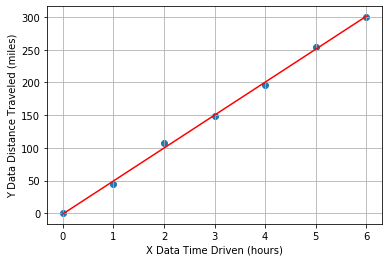In :
# Compute the total change in distance and change in time
total_distance = distances[-1] - distances
total_time = times[-1] - times

# Estimate the slope of the data from the ratio of the changes
average_speed = total_distance / total_time

# Predict the distance traveled for a time not measured
elapse_time = 2.5
distance_traveled = average_speed * elapse_time

print(f"Total Distance {total_distance}")
print(f"\nTotal Time {total_time}")
print(f"\nAverage Speed {average_speed}")
print(f"\nThe distance traveled is {distance_traveled}")

Total Distance 300.0

Total Time 6.0

Average Speed 50.0

The distance traveled is 125.0


Notice that the answer distance is 'inside' that range of data values, so, less than the max(distances) but greater than the min(distances)

# Reasons for Modeling: Extrapolation¶

Another common use of modeling is extrapolation to estimate data values "outside" or "beyond" the range (min and max values of time) of the measured data. Here, we have measured distances for times $0$ through $5$ hours, but we are interested in estimating how far we'd go in $8$ hours. Using the same data set from the previous exercise, we have prepared a linear model distance = model(time). Use that model() to make a prediction about the distance traveled for a time much larger than the other times in the measurements.

In :
# Plot the observed data
plt.scatter(times, distances)

# Fit a model and predict future dates
predict_vals = [0.0,1.0,2.0,3.0,4.0,5.0,6.0,8.0,9.0,10.0]
model = np.polyfit(times, distances, 1)
predicted = np.polyval(model, predict_vals)

# Plot the model
plt.plot(predict_vals, predicted, color='r')
plt.grid(True)
plt.xlabel('X Data Time Driven (hours)')
plt.ylabel('Y Data Distance Traveled (miles)');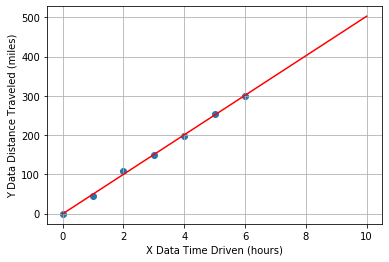In :
def model(time, a0=0, a1=average_speed):
"""
Purpose:
For a given value of time, compute the model value for distance
Args:
time (float, np.ndarray): elapse time in units of hours
a0 (float): default=0, coefficient for the Zeroth order term in the model, i.e. a0 + a1*x
a1 (float): default=50, coefficient for the 1st order term in the model, i.e. a0 + a1*x
Returns:
distance (float, np.ndarray): model values corresponding to input time array, with the same length/size.
"""
distance = a0 + (a1*time)
return distance

In :
# Select a time not measured.
time = 8

# Use the model to compute a predicted distance for that time.
distance = model(time)

# Inspect the value of the predicted distance traveled.
print(distance)

# Determine if you will make it without refueling.

400.0
True


Notice that the car can travel just to the range limit of $400$ miles, so you'd run out of gas just as you completed the trip

# Reasons for Modeling: Estimating Relationships¶

Another common application of modeling is to compare two data sets by building models for each, and then comparing the models. Here, we are given data for a road trip two cars took together. The cars stopped for gas every $50$ miles, but each car did not need to fill up the same amount, because the cars do not have the same fuel efficiency (MPG). The function efficiency_model(miles, gallons) is created to estimate efficiency as average miles traveled per gallons of fuel consumed.

## Data¶

In :
car1 = {'miles': [1. , 50.9, 100.8, 150.7, 200.6, 250.5, 300.4, 350.3, 400.2, 450.1,500.],
'gallons': [0.03333333, 1.69666667, 3.36, 5.02333333, 6.68666667, 8.35, 10.01333333, 11.67666667,
13.34, 15.00333333,  16.66666667]}

car2 = {'miles': [1. , 50.9, 100.8, 150.7, 200.6, 250.5, 300.4, 350.3, 400.2, 450.1, 500. ],
'gallons': [0.02 ,  1.018, 2.016, 3.014, 4.012, 5.01, 6.008, 7.006, 8.004, 9.002, 10. ]}


## Plot Data¶

In :
plt.scatter(car1['miles'], car1['gallons'], label='car1')
plt.scatter(car2['miles'], car2['gallons'], label='car2')
plt.grid(True)
plt.ylabel('Y Data Fuel Consumed (gallons)')
plt.xlabel('X Data Distance Traveled (miles)')
plt.legend();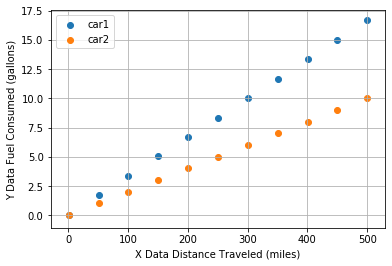In :
# Complete the function to model the efficiency.
def efficiency_model(miles, gallons):
return np.mean(miles/gallons)

# Use the function to estimate the efficiency for each car.
car1['mpg'] = efficiency_model(np.array(car1['miles']), np.array(car1['gallons']))
car2['mpg'] = efficiency_model(np.array(car2['miles']), np.array(car2['gallons']))

# Finish the logic statement to compare the car efficiencies.
if car1['mpg'] > car2['mpg'] :
print('car1 is the best')
elif car1['mpg'] < car2['mpg'] :
print('car2 is the best')
else:
print('the cars have the same efficiency')

car2 is the best


Notice the original plot that visualized the raw data, the slope is $1$/MPG and so car1 is steeper than car2, but if you plot(gallons, miles) the slope is MPG, and so car2 has a steeper slope than car1

In :
plt.scatter(car1['gallons'], car1['miles'], label='car1')
plt.scatter(car2['gallons'], car2['miles'], label='car2')
plt.grid(True)
plt.xlabel('Y Data Fuel Consumed (gallons)')
plt.ylabel('X Data Distance Traveled (miles)')
plt.legend();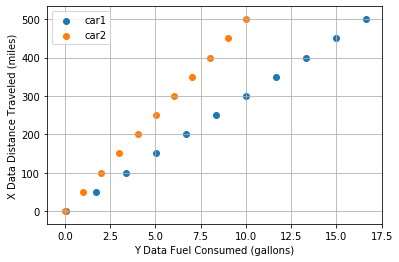# Mean, Deviation, & Standard Deviation¶

The mean describes the center of the data. The standard deviation describes the spread of the data. But to compare two variables, it is convenient to normalize both. Here, we are provided with two arrays of data, which are highly correlated, and we will compute and visualize the normalized deviations of each array.

## Create Data¶

In :
num_samples = 50

# The desired mean values of the sample.
mu = [6.01327144, 301.09351247]

# The desired covariance matrix.
cov = [[1.44408412e+00, 7.11018554e+01],
[7.11018554e+01, 3.62713035e+03]]

# Generate the random samples.
Y = np.random.multivariate_normal(mu, cov, size=num_samples)

# x and y data
x = Y[:,0]
y = Y[:,1]

In :
# Compute the deviations by subtracting the mean offset
dx = x - np.mean(x)
dy = y - np.mean(y)

# Normalize the data by dividing the deviations by the standard deviation
zx = dx / np.std(x)
zy = dy / np.std(y)

In :
plt.figure(figsize=(10,1))
plt.plot(dx, color='r')
plt.plot(dy, color='b')
plt.xlabel('Array Index')
plt.ylabel('Deviations of x & y')
plt.show()

plt.figure(figsize=(10,1))
plt.plot(zx, color='r')
plt.plot(zy, color='b')
plt.xlabel('Array Index')
plt.ylabel('Normalized Deviations of x & y')
plt.show()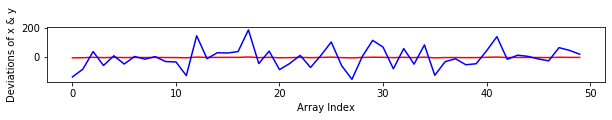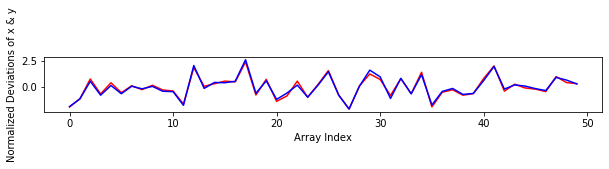Notice how hard it is to compare $dx$ and $dy$, versus comparing the normalized $zx$ and $zy$.

# Covariance vs Correlation¶

Covariance is a measure of whether two variables change ("vary") together. It is calculated by computing the products, point-by-point, of the deviations seen in the previous exercise, dx[n]*dy[n], and then finding the average of all those products.

Correlation is in essence the normalized covariance. Here, we have with two arrays of data, which are highly correlated, and we will visualize and compute both the covariance and the correlation.

In :
# Compute the covariance from the deviations.
dx = x - np.mean(x)
dy = y - np.mean(y)
covariance = np.mean(dx * dy)
print("Covariance: ", covariance)

# Compute the correlation from the normalized deviations.
zx = dx / np.std(x)
zy = dy / np.std(y)
correlation = np.mean(zx * zy)
print("Correlation: ", correlation)

Covariance:  98.65684086428189
Correlation:  0.9871569534589

In :
plt.plot(zx*zy)
plt.axhline(0, linestyle='dashed', color='r')
plt.xlabel('Array Index')
plt.ylabel('Product of Normalized Deviations')
plt.title(f'Correlation = np.mean(zx*zy) = {round(correlation,2)*100}');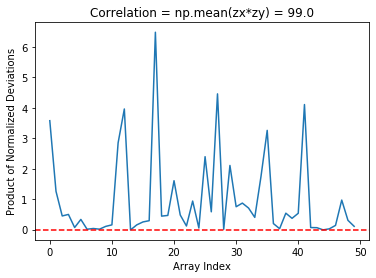Notice that we've plotted the product of the normalized deviations, and labeled the plot with the correlation, a single value that is the mean of that product. The product is always positive and the mean is typical of how the two vary together.

# Correlation Strength¶

Intuitively, we can look at the plots provided and "see" whether the two variables seem to "vary together".

• Data Set A: $x$ and $y$ change together and appear to have a strong relationship.
• Data Set B: there is a rough upward trend; $x$ and $y$ appear only loosely related.
• Data Set C: looks like random scatter; $x$ an $y$ do not appear to change together and are unrelated.

## Create Data¶

In :
# Generate correlated random variables

# The desired number of samples
num_samples = 50

# The desired mean values of the sample.
mu = [4.71815762, 9.44076045, 5.09321393, 10.16904155, 5.0165893, 10.6883259]

# The desired covariance matrix.
cov = [[1.36208968, 2.70163528, 1.37835545, 2.78133499, 1.73359544, 0.95056748],
[2.70163528, 5.40596017, 2.73195338, 5.65673871, 3.43406231, 2.30481703],
[1.37835545, 2.73195338, 1.47904869, 3.23872554, 1.77708497, 1.88996037],
[2.78133499, 5.65673871, 3.23872554, 24.2142715, 3.65117281, 14.5615414],
[1.73359544, 3.43406231, 1.77708497, 3.65117281, 2.25638144, 2.41304719],
[0.95056748, 2.30481703, 1.88996037, 14.5615414, 2.41304719, 295.880271]]

# Generate the random samples.
y = np.random.multivariate_normal(mu, cov, size=num_samples)

# Create a dictionaty from the random samples split into 3 sets each with an x and y array of values
data_sets = {'A': {'x':y[:,0],
'y':y[:,1]},
'B': {'x':y[:,2],
'y':y[:,3]},
'C': {'x':y[:,4],
'y':y[:,5]}}


Recall that deviations differ from the mean, and we normalized by dividing the deviations by standard deviation. Here we will compare the $3$ data sets by computing correlation, and determining which data set has the most strongly correlated variables $x$ and $y$.

In :
for name, data in data_sets.items():
plt.scatter(data['x'], data['y'], color='k')
plt.axhline(0, linestyle='dashed', color='b')
plt.grid(True)
plt.xlabel('X')
plt.ylabel('Y')
plt.title(f'Data set {name} has correlation = ?')
plt.show()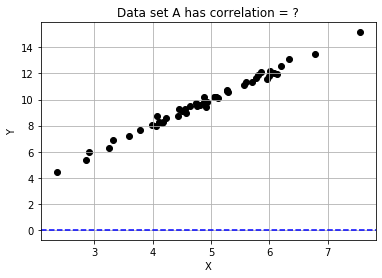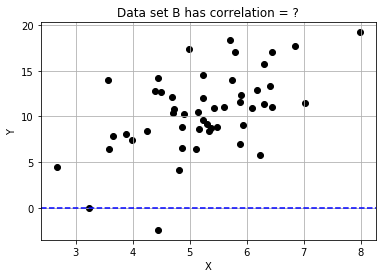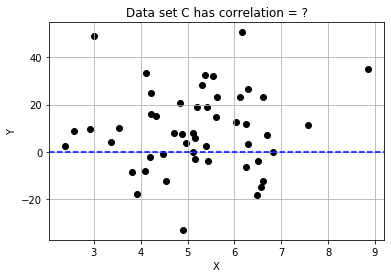In :
# Complete the function that will compute correlation.
def correlation(x,y):
x_dev = x - np.mean(x)
y_dev = y - np.mean(y)
x_norm = x_dev / np.std(x)
y_norm = y_dev / np.std(y)
return np.mean(x_norm * y_norm)

# Compute and store the correlation for each data set in the list.
for name, data in data_sets.items():
data['correlation'] = correlation(data['x'], data['y'])
print('\ndata set {} has correlation {:.2f}'.format(name, data['correlation']))

data set A has correlation 0.99

data set B has correlation 0.54

data set C has correlation 0.06

In :
# Assign the data set with the best correlation.
best_data = data_sets['A']


Note that the strongest relationship is in Dataset $A$, with correlation closest to $1.0$ and the weakest is Datatset $C$ with correlation value closest to zero.

## Taylor Series¶

The Taylor series of a function is an infinite sum of terms, where each term has a larger exponent like x, x2, x3, etc. A Taylor Series is expressed in terms of the function's derivatives at a single point. For most common functions, the function and the sum of its Taylor series are equal near this point. Taylor's series are named after Brook Taylor who introduced them in $1715$.

1. approximate any curve
2. polynomial form
3. often, first order is enough
• The derivative of a function of a real variable measures the sensitivity to change of the function value (output value) with respect to a change in its argument (input value). Derivatives are a fundamental tool of calculus. For example, the derivative of the position of a moving object with respect to time is the object's velocity: this measures how quickly the position of the object changes when time advances.
• A polynomial in a single indeterminate $x$ can always be written (or rewritten) in the form
$$a_{n}x^{n}+a_{n-1}x^{n-1}+\dots +a_{2}x^{2}+a_{1}x+a_{0},$$
• where $a_{0},\ldots ,a_{n}$ are constants and $x$ is the indeterminate. The word "indeterminate" means that $x$ represents no particular value, although any value may be substituted for it. The mapping that associates the result of this substitution to the substituted value is a function, called a polynomial function.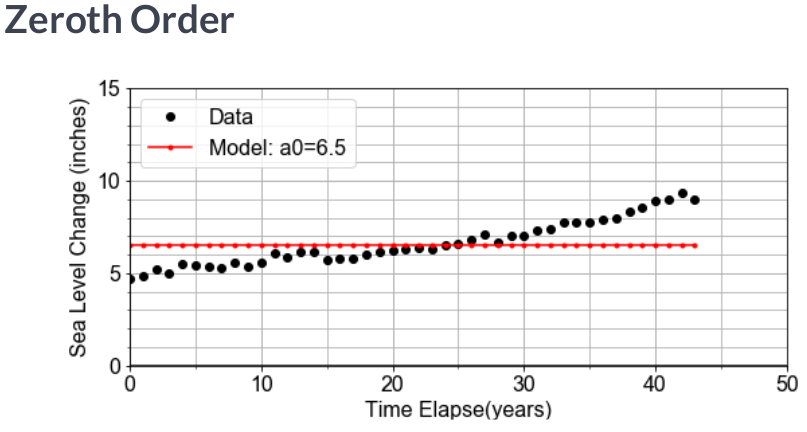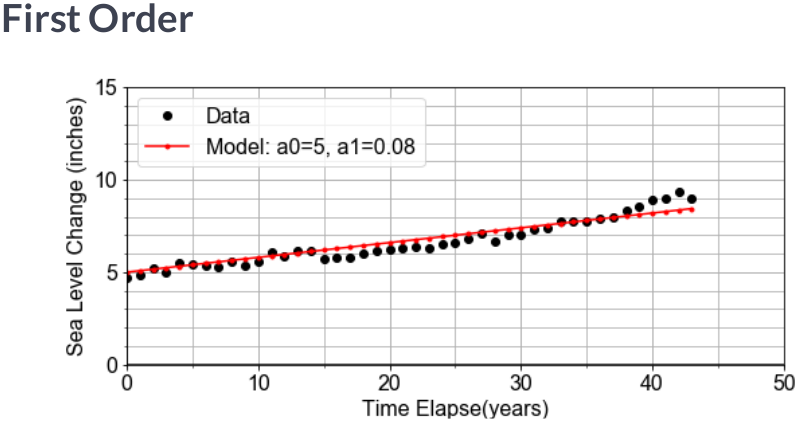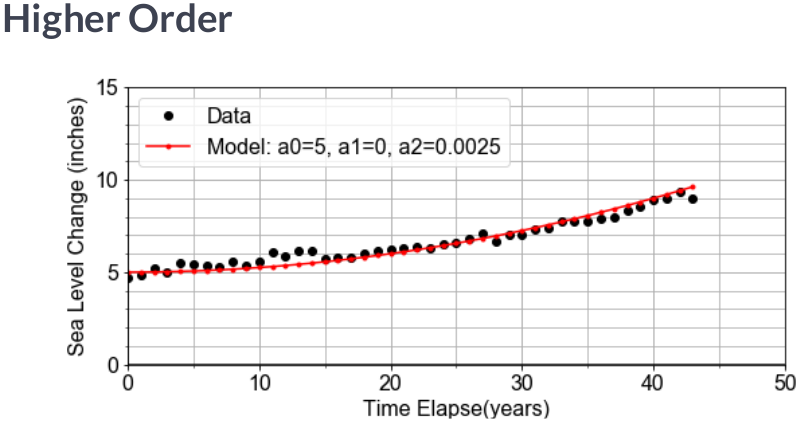# Model Components¶

Previously, we have been given a pre-defined model to work with. In this Here, we will implement a model function that returns model values for $y$, computed from input $x$ data, and any input coefficients for the "zero-th" order term a0, the "first-order" term a1, and a quadratic term a2 of a model.

$$y = a_0 + a_1 x + a_2 x^2$$

Recall that "first order" is linear, so we'll set the defaults for this general linear model with a2=0, but later, we will change this for comparison.

In :
# Define the general model as a function
def model(x, a0=3, a1=2, a2=0):
return a0 + (a1*x) + (a2*x*x)

# Generate array x, then predict y values for specific, non-default a0 and a1
x = np.linspace(-10, 10, 21)
y = model(x)

plt.scatter(x, y)
plt.plot(x, np.poly1d(np.polyfit(x, y, 1))(x), color='r')
plt.grid(True)
plt.title('Plot of modeled Y for given data X')
plt.xlabel('X')
plt.ylabel('Y');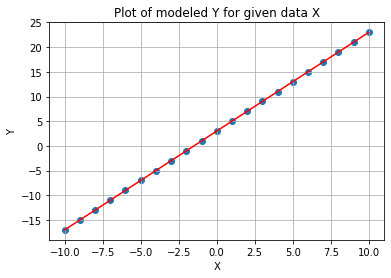Notice that we used model() to compute predicted values of y for given possibly measured values of x. The model takes the independent data and uses it to generate a model for the dependent variables corresponding values.

# Model Parameters¶

Now that we've built a general model, let's "optimize" or "fit" it to a new measured data set, xd, yd, by finding the specific values for model parameters a0, a1 for which the model data and the measured data line up on a plot.

This is an iterative visualization strategy, where we start with a guess for model parameters, pass them into the model(), over-plot the resulting modeled data on the measured data, and visually check that the line passes through the points. If it doesn't, we change the model parameters and try again.

In :
df_xy = pd.read_csv('df_xy.csv').drop('Unnamed: 0', axis=1)
df_xy.info()

xd = df_xy['X'].values
yd = df_xy['Y'].values

<class 'pandas.core.frame.DataFrame'>
RangeIndex: 21 entries, 0 to 20
Data columns (total 2 columns):
X    21 non-null float64
Y    21 non-null float64
dtypes: float64(2)
memory usage: 464.0 bytes


### Plot Data¶

In :
plt.scatter(xd, yd)
plt.grid(True)
plt.xlim(-5,15)
plt.ylim(-250,750)
plt.axvline(0, color='k')
plt.axhline(0, color='k')
plt.title('Hiking Trip')
plt.xlabel('Step distance (km)')
plt.ylabel('Altitude (meters)');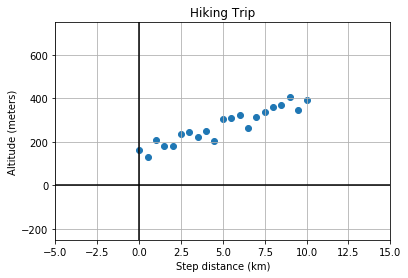In :
# Complete the plotting function definition
def plot_data_with_model(xd, yd, ym):
plt.scatter(xd, yd)
plt.grid(True)
plt.xlim(-5,15)
plt.ylim(-250,750)
plt.axvline(0, color='k')
plt.axhline(0, color='k')
plt.title('Hiking Trip')
plt.xlabel('Step distance (km)')
plt.ylabel('Altitude (meters)');
plt.plot(xd, ym, color='red')  # over-plot modeled y data
plt.show()
return

# Select new model parameters a0, a1, and generate modeled ym from them.
a0 = 150
a1 = 25
ym = model(xd, a0, a1)

# Plot the resulting model to see whether it fits the data
fig = plot_data_with_model(xd, yd, ym)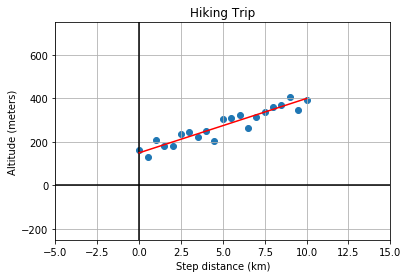Notice again that the measured x-axis data xd is used to generate the modeled y-axis data ym so to plot the model, you are plotting ym vs xd, which may seem counter-intuitive at first. But we are modeling the $y$ response to a given $x$; we are not modeling $x$.

# Linear Proportionality¶

The definition of temperature scales is related to the linear expansion of certain liquids, such as mercury and alcohol. Originally, these scales were literally rulers for measuring length of fluid in the narrow marked or "graduated" tube as a proxy for temperature. The alcohol starts in a bulb, and then expands linearly into the tube, in response to increasing temperature of the bulb or whatever surrounds it.

Here, we will explore the conversion between the Fahrenheit and Celsius temperature scales as a demonstration of interpreting slope and intercept of a linear relationship within a physical context.

In :
# Complete the function to convert C to F
def convert_scale(temps_C):
(freeze_C, boil_C) = (0, 100)
(freeze_F, boil_F) = (32, 212)
change_in_C = boil_C - freeze_C
change_in_F = boil_F - freeze_F
slope = change_in_F / change_in_C
intercept = freeze_F - freeze_C
temps_F = intercept + (slope * temps_C)
return temps_F

# Use the convert function to compute values of F and plot them
temps_C = np.linspace(0, 100, 101)
temps_F = convert_scale(temps_C)

plt.plot(temps_C, temps_F)
plt.grid(True)
plt.ylabel('Temperature (Fahrenheit)')
plt.xlabel('Temperature (Celsius)');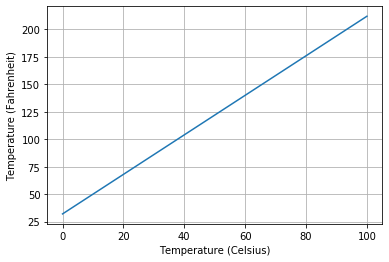# Rate of Change (ROC)¶

The rate of change - ROC - is the speed at which a variable changes over a specific period of time. ROC is often used when speaking about momentum, and it can generally be expressed as a ratio between a change in one variable relative to a corresponding change in another; graphically, the rate of change is represented by the slope of a line. The ROC is often illustrated by the Greek letter delta $\Delta$.

# Slope and Rates-of-Change¶

Here, we will model the motion of a car driving (roughly) constant velocity by computing the average velocity over the entire trip. The linear relationship modeled is between the time elapsed and the distance traveled.

In this case, the model parameter a1, or slope, is approximated or "estimated", as the mean velocity, or put another way, the "rate-of-change" of the distance ("rise") divided by the time ("run").

In :
df_td = pd.read_csv('time_distance.csv').drop('Unnamed: 0', axis=1)
df_td.info()

times = df_td['times']
distances = df_td['distances']

<class 'pandas.core.frame.DataFrame'>
RangeIndex: 25 entries, 0 to 24
Data columns (total 2 columns):
times        25 non-null float64
distances    25 non-null float64
dtypes: float64(2)
memory usage: 528.0 bytes

In :
# Compute an array of velocities as the slope between each point
diff_distances = np.diff(distances)
diff_times = np.diff(times)
velocities = diff_distances / diff_times

# Chracterize the center and spread of the velocities
v_avg = np.mean(velocities)
v_max = np.max(velocities)
v_min = np.min(velocities)
v_range = v_max - v_min

In :
plt.scatter(times[1:], velocities, label='Velocities')
plt.axhline(v_avg, color='r', lw=5, alpha=0.4, label='Mean velocity')
plt.ylim(0,100)
plt.grid(True)
plt.ylabel('Instantaneous velocity (Kilometers/Hours)')
plt.xlabel('Time (hours)')
plt.legend();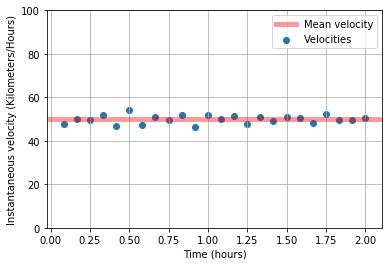Generally we might use the average velocity as the slope in our model. But notice that there is some random variation in the instantaneous velocity values when plotted as a time series. The range of values v_max - v_min is one measure of the scale of that variation, and the standard deviation of velocity values is another measure.

# Ordinary least squares¶

In statistics, ordinary least squares (OLS) is a type of linear least squares method for estimating the unknown parameters in a linear regression model. OLS chooses the parameters of a linear function of a set of explanatory variables by the principle of least squares: minimizing the sum of the squares of the differences between the observed dependent variable (values of the variable being observed) in the given dataset and those predicted by the linear function.

Simple linear regression model

If the data matrix $X$ contains only two variables, a constant and a scalar regressor $x_i$, then this is called the "simple regression model". It provides much simpler formulas even suitable for manual calculation. The parameters are commonly denoted as ($\alpha$, $\beta$):

$$y_{i}=\alpha +\beta x_{i}+\varepsilon _{i}$$

The least squares estimates in this case are given by simple formulas

{\begin{aligned}{\hat {\beta }}&={\frac {\sum {x_{i}y_{i}}-{\frac {1}{n}}\sum {x_{i}}\sum {y_{i}}}{\sum {x_{i}^{2}}-{\frac {1}{n}}(\sum {x_{i}})^{2}}}={\frac {\operatorname {Cov} [x,y]}{\operatorname {Var} [x]}}\\{\hat {\alpha }}&={\overline {y}}-{\hat {\beta }}\,{\overline {x}}\ ,\end{aligned}}

where Var(.) and Cov(.) are sample parameters.

# Intercept and Starting Points¶

Here, we will see the intercept and slope parameters in the context of modeling measurements taken of the volume of a solution contained in a large glass jug. The solution is composed of water, grains, sugars, and yeast. The total mass of both the solution and the glass container was also recorded, but the empty container mass was not noted.

Our job is to use the pandas DataFrame df, with data columns volumes and masses, to build a linear model that relates the masses (y-data) to the volumes (x-data). The slope will be an estimate of the density (change in mass / change in volume) of the solution, and the intercept will be an estimate of the empty container weight (mass when volume=$0$).

In :
fpath_csv = 'https://assets.datacamp.com/production/repositories/1480/datasets/e4c4cdd076de27d3c1bccfe1d0019d279c08d2fc/solution_data.csv'
print(df.info())

<class 'pandas.core.frame.DataFrame'>
RangeIndex: 101 entries, 0 to 100
Data columns (total 2 columns):
volumes    101 non-null float64
masses     101 non-null float64
dtypes: float64(2)
memory usage: 1.7 KB
None
volumes    masses
0      2.0  7.812435
1      2.1  7.698824
2      2.2  7.817183
3      2.3  7.872703
4      2.4  8.176541

In :
# Import ols from statsmodels, and fit a model to the data
from statsmodels.formula.api import ols
model_fit = ols(formula="masses ~ volumes", data=df)
model_fit = model_fit.fit()

# Extract the model parameter values, and assign them to a0, a1
a0 = model_fit.params['Intercept']
a1 = model_fit.params['volumes']

# Print model parameter values with meaningful names, and compare to summary()
print( "container_mass   = {:0.4f}".format(a0) )
print( "\nsolution_density = {:0.4f}".format(a1) )
print('\n',model_fit.summary())

container_mass   = 5.4349

solution_density = 1.1029

OLS Regression Results
==============================================================================
Dep. Variable:                 masses   R-squared:                       0.999
Method:                 Least Squares   F-statistic:                 1.328e+05
Date:                Thu, 04 Jun 2020   Prob (F-statistic):          1.19e-156
Time:                        19:46:18   Log-Likelihood:                 102.39
No. Observations:                 101   AIC:                            -200.8
Df Residuals:                      99   BIC:                            -195.5
Df Model:                           1
Covariance Type:            nonrobust
==============================================================================
coef    std err          t      P>|t|      [0.025      0.975]
------------------------------------------------------------------------------
Intercept      5.4349      0.023    236.805      0.000       5.389       5.480
volumes        1.1029      0.003    364.408      0.000       1.097       1.109
==============================================================================
Omnibus:                        0.319   Durbin-Watson:                   2.072
Prob(Omnibus):                  0.852   Jarque-Bera (JB):                0.169
Skew:                           0.100   Prob(JB):                        0.919
Kurtosis:                       3.019   Cond. No.                         20.0
==============================================================================

Warnings:
 Standard Errors assume that the covariance matrix of the errors is correctly specified.


# Residual in a Regression Model¶

A residual is the vertical distance between a data point and the regression line. Each data point has one residual. They are positive if they are above the regression line and negative if they are below the regression line. If the regression line actually passes through the point, the residual at that point is zero.

## Errors and residuals¶

The error (or disturbance) of an observed value is the deviation of the observed value from the (unobservable) true value of a quantity of interest (for example, a population mean), and the residual of an observed value is the difference between the observed value and the estimated value of the quantity of interest (for example, a sample mean). The distinction is most important in regression analysis, where the concepts are sometimes called the regression errors and regression residuals

### Residual Sum of Squares (RSS)¶

The residual sum of squares measures the amount of error remaining between the regression function and the data set. A smaller residual sum of squares figure represents a regression function. Residual sum of squares–also known as the sum of squared residuals–essentially determines how well a regression model explains or represents the data in the model.

$$SS_{\text{res}}=\sum _{i}(y_{i}-f_{i})^{2}=\sum _{i}e_{i}^{2}\$$

# Residual Sum of the Squares¶

Previously we saw that the altitude along a hiking trail was roughly fit by a linear model, and we introduced the concept of differences between the model and the data as a measure of model goodness.

Here, we'll work with the same measured data, and quantifying how well a model fits it by computing the sum of the square of the "differences", also called "residuals".

In :
# Function to generate x and y data
num_pts=21; a0=3.0*50; a1=0.5*50; mu=0; sigma=1; ae=0.5*50; seed=1234;
np.random.seed(seed)
xmin = 0
xmax = 10
x1 = np.linspace(xmin, xmax, num_pts)
e1 = np.array([np.random.normal(mu, sigma) for n in range(num_pts)])
y1 = a0 + (a1*x1) + ae*e1
return x1, y1

In :
# Load the data

# Model the data with specified values for parameters a0, a1
y_model = model(x_data, a0=150, a1=25)

# Compute the RSS value for this parameterization of the model

RSS = 14444.484117694472


The value we compute for RSS is not meaningful by itself, but later it becomes meaningful in context when we compare it to other values of RSS computed for other parameterizations of the model.

# Minimizing the Residuals¶

Here, we will complete a function to visually compare model and data, and compute and print the RSS. We will call it more than once to see how RSS changes when you change values for a0 and a1. We'll see that the values for the parameters we found earlier are the ones needed to minimize the RSS.

In :
# Complete function to load data, build model, compute RSS, and plot
ym = model(xd, a0, a1)
residuals = ym - yd
plt.scatter(xd, yd)
plt.grid(True)
plt.xlim(-5,15)
plt.ylim(-250,750)
plt.axvline(0, color='k')
plt.axhline(0, color='k')
plt.title(summary)
plt.xlabel('Step distance (km)')
plt.ylabel('Altitude (meters)')
plt.plot(xd, ym, color='red')  # over-plot modeled y data
plt.show()

# Chose model parameter values and pass them into RSS function
print(summary)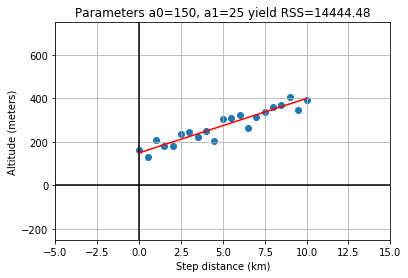Parameters a0=150, a1=25 yield RSS=14444.48


As stated earlier, the significance of RSS is in context of other values. More specifically, the minimum RSS is of value in identifying the specific set of parameter values for our model which yield the smallest residuals in an overall sense.

Here we will compute and visualize how RSS varies for different values of model parameters. Start by holding the intercept constant, but vary the slope: and for each slope value, we'll compute the model values, and the resulting RSS. Once you have an array of RSS values, we will determine minimal RSS value, in code, and from that minimum, determine the slope that resulted in that minimal RSS.

## Data¶

In :
x_data = df_xy['X'].values
y_data = df_xy['Y'].values

In :
# Function to compute RSS

In :
# Empty list for RSS array

# Loop over all trial values in a1_array, computing rss for each
a1_array = np.linspace(15, 35, 101)
for a1_trial in a1_array:
y_model = model(x_data, a0=150, a1=a1_trial)

# Find the minimum RSS and the a1 value from whence it came

plt.grid(True)
plt.xlabel('Slope a1')

The minimum RSS = 14411.193018382088, came from a1 = 24.8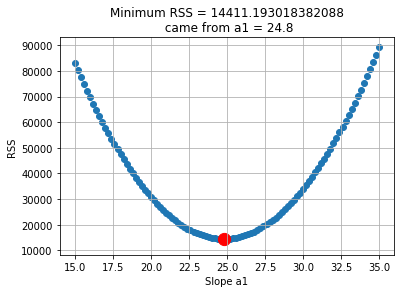The best slope is the one out of an array of slopes than yielded the minimum RSS value out of an array of RSS values. Python tip: notice that we started with rss_list to make it easy to .append() but then later converted tonumpy.array() to gain access to all the numpy methods.

# Least-Squares with numpy¶

The formulae below are the result of working through the calculus. Here, we'll trust that the calculus correct, and implement these formulae in code using numpy.

$$a_1=\frac{covariance(x,y)}{variance(x)}$$$$a_0=mean(y)−a_1mean(x)$$

## Data¶

In :
x = df_xy['X'].values
y = df_xy['Y'].values

In :
# prepare the means and deviations of the two variables
x_mean = np.mean(x)
y_mean = np.mean(y)
x_dev = x - x_mean
y_dev = y - y_mean

# Complete least-squares formulae to find the optimal a0, a1
a1 = np.sum(x_dev * y_dev) / np.sum( np.square(x_dev) )
a0 = y_mean - (a1 * x_mean)

# Use the those optimal model parameters a0, a1 to build a model
y_model = model(np.array(x), a0, a1)

# plot to verify that the resulting y_model best fits the data y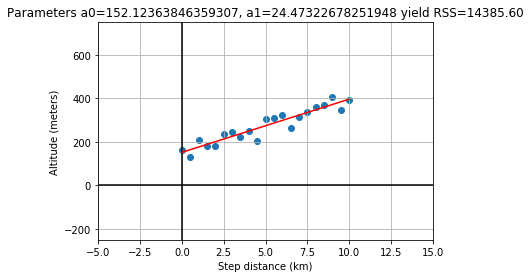Notice that the optimal slope a1, according to least-squares, is a ratio of the covariance to the variance. Also, note that the values of the parameters obtained here are NOT exactly the ones used to generate the pre-loaded data (a1=$25$ and a0=$150$), but they are close to those. Least-squares does not guarantee zero error; there is no perfect solution, but in this case, least-squares is the best we can do.

# Optimization with Scipy¶

It is possible to write a numpy implementation of the analytic solution to find the minimal RSS value. But for more complex models, finding analytic formulae is not possible, and so we turn to other methods.

Here we will use scipy.optimize to employ a more general approach to solve the same optimization problem.

In so doing, we will see additional return values from the method that tell answer us "how good is best". Here we will use the same measured data and parameters as seen in the last exercise for ease of comparison of the new scipy approach.

In :
from scipy import optimize

# Define a model function needed as input to scipy
def model_func(x, a0, a1):
return a0 + (a1*x)

# Load the measured data you want to model

# call curve_fit, passing in the model function and data; then unpack the results
param_opt, param_cov = optimize.curve_fit(model_func, x_data, y_data)
a0 = param_opt  # a0 is the intercept in y = a0 + a1*x
a1 = param_opt  # a1 is the slope     in y = a0 + a1*x

# test that these parameters result in a model that fits the data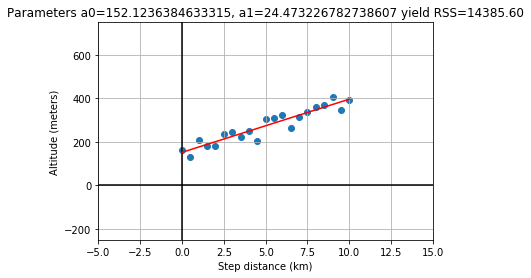Notice that we passed the function object itself, model_func into curve_fit, rather than passing in the model data. The model function object was the input, because the optimization wants to know what form in general it's solve for; had we passed in a model_func with more terms like an a2*x**2 term, we would have seen different results for the parameters output.

# Least-Squares with statsmodels¶

Several python libraries provide convenient abstracted interfaces so that you need not always be so explicit in handling the machinery of optimization of the model.

As an example, in this exercise, we will use the statsmodels library in a more high-level, generalized work-flow for building a model using least-squares optimization (minimization of RSS).

In :
x_data, y_data = load_data()
df = pd.DataFrame(dict(x_column=x_data, y_column=y_data))

# Pass data and formula into ols(), use and .fit() the model to the data
model_fit = ols(formula="y_column ~ x_column", data=df).fit()

# Use .predict(df) to get y_model values, then over-plot y_data with y_model
y_model = model_fit.predict(df)

# Extract the a0, a1 values from model_fit.params
a0 = model_fit.params['Intercept']
a1 = model_fit.params['x_column']

# Visually verify that these parameters a0, a1 give the minimum RSS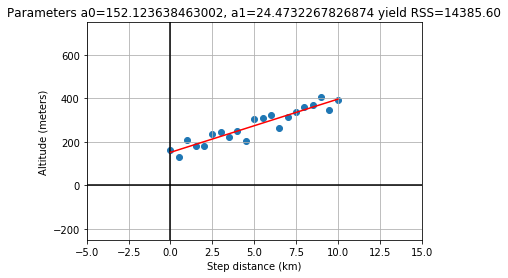Note that the params container always uses 'Intercept' for the a0 key, but all higher order terms will have keys that match the column name from the pandas DataFrame that we passed into ols().

# Linear Model in Anthropology¶

If you found part of a skeleton, from an adult human that lived thousands of years ago, how could you estimate the height of the person that it came from? This exercise is in part inspired by the work of forensic anthropologist Mildred Trotter, who built a regression model for the calculation of stature estimates from human "long bones" or femurs that is commonly used today.

In this exercise, we'll use data from many living people, and the python library scikit-learn, to build a linear model relating the length of the femur (thigh bone) to the "stature" (overall height) of the person. Then, we'll apply our model to make a prediction about the height of an ancient ancestor.

In :
fpath_csv = 'https://assets.datacamp.com/production/repositories/1480/datasets/a1871736d7829e85ec4ead2212d621df69bb3977/femur_data.csv'
df.info()

legs = df['length'].values
heights = df['height'].values

<class 'pandas.core.frame.DataFrame'>
RangeIndex: 21 entries, 0 to 20
Data columns (total 2 columns):
length    21 non-null float64
height    21 non-null float64
dtypes: float64(2)
memory usage: 464.0 bytes


### Plot¶

In :
plt.scatter(df['length'], df['height'])
plt.grid(True)
plt.xlim(-10,100)
plt.ylim(-30,300)
plt.axvline(0, color='k')
plt.axhline(0, color='k')
plt.title('Relating long bone length and hieght')
plt.xlabel('Femur Length (cm)')
plt.ylabel('Height (cm)');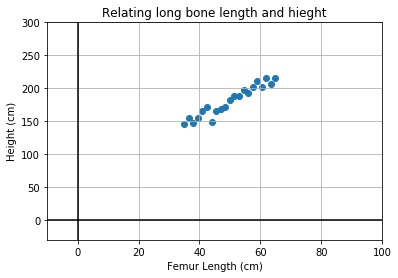In :
# import the sklearn class LinearRegression and initialize the model
from sklearn.linear_model import LinearRegression
model = LinearRegression(fit_intercept=False)

# Prepare the measured data arrays and fit the model to them
legs = legs.reshape(len(legs),1)
heights = heights.reshape(len(heights),1)
model.fit(legs, heights)

# Use the fitted model to make a prediction for the found femur
fossil_leg = [[50.7]]
fossil_height = model.predict(fossil_leg)
print("Predicted fossil height = {:0.2f} cm".format(fossil_height[0,0]))

Predicted fossil height = 181.34 cm


Notice that we used the pre-loaded data to fit or "train" the model, and then applied that model to make a prediction about newly collected data that was not part of the data used to fit the model. Also notice that model.predict() returns the answer as an array of shape = (1,1), so we had to index into it with the [0,0] syntax when printing.

# Linear Model in Oceanography¶

Time-series data provides a context in which the "slope" of the linear model represents a "rate-of-change".

In this exercise, we will use measurements of sea level change from $1970$ to $2010$, build a linear model of that changing sea level and use it to make a prediction about the future sea level rise.

In :
fpath_csv = 'https://assets.datacamp.com/production/repositories/1480/datasets/0c1231fa1e777d3d7dd2d0ab877158a0dd219a5b/sea_level_data.csv'
df0.info()

years = df0['year'].values
levels = df0['sea_level_inches'].values

<class 'pandas.core.frame.DataFrame'>
RangeIndex: 44 entries, 0 to 43
Data columns (total 2 columns):
year                44 non-null int64
sea_level_inches    44 non-null float64
dtypes: float64(1), int64(1)
memory usage: 832.0 bytes


### Plot¶

In :
plt.scatter(df0['year'], df0['sea_level_inches'])
plt.grid(True)
plt.title('Global Average Seal Level Change')
plt.xlabel('Seal Level Change (inches)')
plt.ylabel('Time (years)');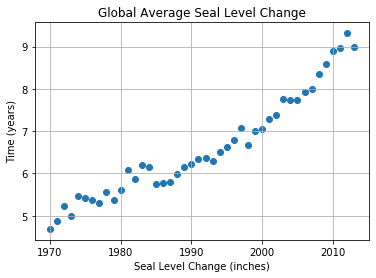In :
# Prepare the measured data arrays and fit the model to them
years = years.reshape(len(years),1)
levels = levels.reshape(len(years),1)

# Build a model, fit to the data
model = LinearRegression(fit_intercept=True)
model.fit(years, levels)

# Use model to make a prediction for one year, 2100
future_year = 2100
future_level = model.predict([[future_year]])
print("Prediction: year = {}, level = {:.02f}".format(future_year, future_level[0,0]))

# Use model to predict for many years, and over-plot with measured data
years_forecast = np.linspace(1970, 2100, 131).reshape(-1, 1)
levels_forecast = model.predict(years_forecast)

plt.scatter(df0['year'], df0['sea_level_inches'])
plt.plot(years_forecast, levels_forecast, color='r')
plt.grid(True)
plt.title('Global Average Seal Level Change')
plt.xlabel('Seal Level Change (inches)')
plt.ylabel('Time (years)');

Prediction: year = 2100, level = 16.66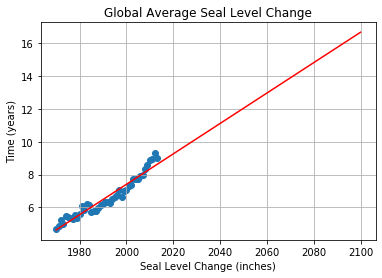Notice also that although our model is linear, the actual data appears to have an up-turn that might be better modeled by adding a quadratic or even exponential term to our model. The linear model forecast may be underestimating the rate of increase in sea level.

# Linear Model in Cosmology¶

Less than $100$ years ago, the universe appeared to be composed of a single static galaxy, containing perhaps a million stars. Today we have observations of hundreds of billions of galaxies, each with hundreds of billions of stars, all moving.

The beginnings of the modern physical science of cosmology came with the publication in 1929 by Edwin Hubble that included use of a linear model.

In this exercise, we will build a model whose slope will give Hubble's Constant, which describes the velocity of galaxies as a linear function of distance from Earth.

In :
fpath_csv = 'https://assets.datacamp.com/production/repositories/1480/datasets/fffe3864d24c1864a5df0f0985a11d07bc1cd526/hubble_data.csv'
df.info()

<class 'pandas.core.frame.DataFrame'>
RangeIndex: 24 entries, 0 to 23
Data columns (total 3 columns):
names         24 non-null object
distances     24 non-null float64
velocities    24 non-null int64
dtypes: float64(1), int64(1), object(1)
memory usage: 704.0+ bytes


### Plot¶

In :
plt.scatter(df['distances'], df['velocities'])
plt.grid(True)
plt.xlim(-1.00,4.00)
plt.ylim(-400,1200)
plt.xlabel('Distances')
plt.ylabel('Velocities');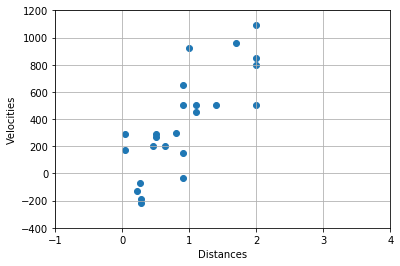In :
# Fit the model, based on the form of the formula
model_fit = ols(formula="velocities ~ distances", data=df).fit()

# Extract the model parameters and associated "errors" or uncertainties
a0 = model_fit.params['Intercept']
a1 = model_fit.params['distances']
e0 = model_fit.bse['Intercept']
e1 = model_fit.bse['distances']

# Print the results
print('For slope a1={:.02f}, the uncertainty in a1 is {:.02f}'.format(a1, e1))
print('For intercept a0={:.02f}, the uncertainty in a0 is {:.02f}'.format(a0, e0))

For slope a1=454.16, the uncertainty in a1 is 75.24
For intercept a0=-40.78, the uncertainty in a0 is 83.44


# Interpolation: Inbetween Times¶

In this exercise, we will build a linear model by fitting monthly time-series data for the Dow Jones Industrial Average (DJIA) and then use that model to make predictions for daily data (in effect, an interpolation). Then we will compare that daily prediction to the real daily DJIA data.

A few notes on the data. "OHLC" stands for "Open-High-Low-Close", which is usually daily data, for example the opening and closing prices, and the highest and lowest prices, for a stock in a given day. DayCount is an integer number of days from start of the data collection.

In :
from pandas_datareader import data as web
import warnings
warnings.filterwarnings('ignore')

start = pd.Timestamp('2013')
end = pd.Timestamp('2015-01-01')

df_daily = web.DataReader('DJIA', 'yahoo', start, end)[['Close']]

# Resample to monthly
df_monthly = df_daily.resample('M').last()

# Count number of days elapsed since a certain date
basedate_d = df_daily.index
df_daily['DayCount'] = df_daily.apply(lambda x: (x.name - basedate_d).days, axis=1)

basedate_m = df_monthly.index
df_monthly['DayCount'] = df_monthly.apply(lambda x: (x.name - basedate_m).days, axis=1)


                   Close  DayCount
Date
2013-01-02  13412.549805         0
2013-01-03  13391.360352         1
2013-01-04  13435.209961         2
2013-01-07  13384.290039         5
2013-01-08  13328.849609         6

Close  DayCount
Date
2013-01-31  13860.580078         0
2013-02-28  14054.490234        28
2013-03-31  14578.540039        59
2013-04-30  14839.799805        89
2013-05-31  15115.570312       120


## Plot Data¶

In :
plt.figure(figsize=(10,5))
plt.scatter(df_monthly.index, df_monthly['Close'], label='Mpnthly Data')
plt.grid(True)
plt.xlabel('Date')
plt.ylabel('DJIA Close ($)') plt.legend();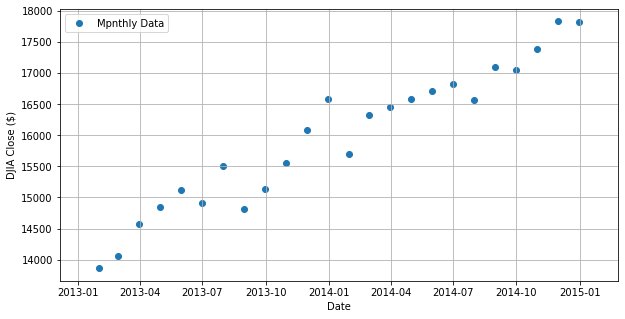In : # fit a model to the df_monthly data model_fit = ols('Close ~ DayCount', data=df_monthly).fit() # Use the model to make a predictions for both monthly and daily data df_monthly['Model'] = model_fit.predict(df_monthly.DayCount) df_daily['Model'] = model_fit.predict(df_daily.DayCount) # Calculate the RSS rss_d = compute_rss(df_daily['Close'], df_daily['Model']) rss_m = compute_rss(df_monthly['Close'], df_monthly['Model']) plt.figure(figsize=(10,5)) plt.scatter(df_monthly.index, df_monthly['Close'], label='Mpnthly Data') plt.plot(df_monthly.index, df_monthly['Model'], label='Model', color='r') plt.grid(True) plt.title(f'RSS {rss_m}') plt.xlabel('Date') plt.ylabel('DJIA Close ($)')
plt.legend();

plt.figure(figsize=(10,5))
plt.scatter(df_daily.index, df_daily['Close'], label='Daily Data')
plt.plot(df_daily.index, df_daily['Model'], label='Model', color='r')
plt.grid(True)
plt.ylabel('DJIA Close ($)') plt.legend();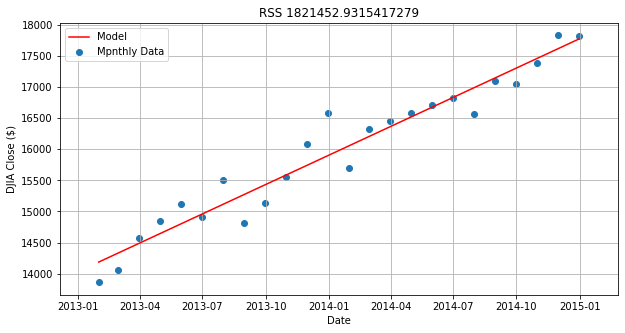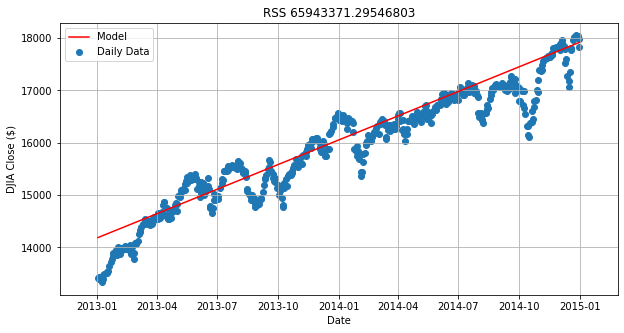Notice the monthly data looked linear, but the daily data clearly has additional, nonlinear trends. Under-sampled data often misses real-world features in the data on smaller time or spatial scales. Using the model from the under-sampled data to make interpolations to the daily data can result is large residuals. Notice that the RSS value for the daily plot is more than 30 times worse than the monthly plot. # Extrapolation: Going Over the Edge¶ In this exercise, we consider the perils of extrapolation. Shown here is the profile of a hiking trail on a mountain. One portion of the trail, marked in black, looks linear, and was used to build a model. But we see that the best fit line, shown in red, does not fit outside the original "domain", as it extends into this new outside data, marked in blue. If we want use the model to make predictions for the altitude, but still be accurate to within some tolerance, what are the smallest and largest values of independent variable x that we can allow ourselves to apply the model to?" In : df = pd.read_csv('hiking.csv').drop('Unnamed: 0', axis=1) df.info() x_data = df['x_data'].values y_data = df['y_data'].values y_model = df['y_model'].values  <class 'pandas.core.frame.DataFrame'> RangeIndex: 61 entries, 0 to 60 Data columns (total 3 columns): x_data 61 non-null float64 y_data 61 non-null float64 y_model 61 non-null float64 dtypes: float64(3) memory usage: 1.6 KB  ## Plot Data¶ In : col = np.where(np.array(x_data)<=0,'k', np.where(np.array(x_data)<10,'b','k')) plt.scatter(x_data, y_data, c=col) plt.plot(x_data, y_model, color='r') plt.grid(True) plt.xlim(-15,25) plt.ylim(-250,750) plt.axvline(0, color='k') plt.axhline(0, color='k') plt.title('Hiking Trip') plt.xlabel('Step distance (kilometers)') plt.ylabel('Altitude (meters)');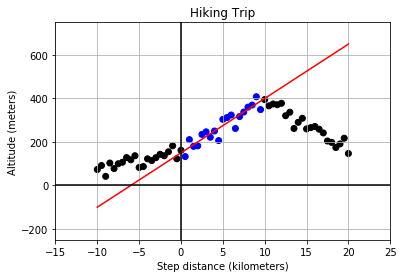In : # Compute the residuals, "data - model", and determine where [residuals < tolerance] residuals = np.abs(y_data - y_model) tolerance = 100 x_good = x_data[residuals < tolerance] # Find the min and max of the "good" values, and plot y_data, y_model, and the tolerance range print(f'Minimum good x value = {np.min(x_good)}') print(f'Maximum good x value = {np.max(x_good)}') col = np.where(np.array(x_data)<=0,'k', np.where(np.array(x_data)<10,'b','k')) plt.scatter(x_data, y_data, c=col) plt.plot(x_data, y_model, color='r') a = x_data <= 13 b = x_data >= -6 mask = (a & b) plt.fill_between(x_data, y_model-tolerance, y_model+tolerance, where=mask, alpha=0.2, color='g') plt.grid(True) plt.xlim(-15,25) plt.ylim(-250,750) plt.axvline(0, color='k') plt.axhline(0, color='k') plt.title('Hiking Trip') plt.xlabel('Step distance (kilometers)') plt.ylabel('Altitude (meters)');  Minimum good x value = -5.0 Maximum good x value = 12.0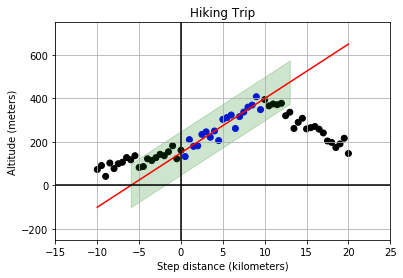# 3 Different R's¶ ## Building Models:¶ • RSS: • Used to help find the optimal values for model parameters • The total sum of squares (proportional to the variance of the data): $$SS_{\text{tot}}=\sum _{i}(y_{i}-{\bar {y}})^{2}$$ • The sum of squares of residuals, also called the residual sum of squares: $$SS_{\text{res}}=\sum _{i}(y_{i}-f_{i})^{2}=\sum _{i}e_{i}^{2}$$ ## Evaluating Models:¶ • RMSE: • Root Mean Square Error (RMSE) is the standard deviation of the residuals (prediction errors). Residuals are a measure of how far from the regression line data points are; RMSE is a measure of how spread out these residuals are. In other words, it tells you how concentrated the data is around the line of best fit. • The RMSE of an estimator$\hat{\theta}$with respect to an estimated parameter$\theta$is defined as the square root of the mean square error: $$\operatorname {RMSE} ({\hat {\theta }})={\sqrt {\operatorname {MSE} ({\hat {\theta }})}}={\sqrt {\operatorname {E} (({\hat {\theta }}-\theta )^{2})}}.$$ • The RMSE of predicted values$\hat y_t$for times$t$of a regression's dependent variable$y_{t}$with variables observed over$T$times, is computed for$T$different predictions as the square root of the mean of the squares of the deviations: $$\operatorname {RMSE} ={\sqrt {\frac {\sum _{t=1}^{T}({\hat {y}}_{t}-y_{t})^{2}}{T}}}.$$ • R-squared • R-squared or the coefficient of determination is a statistical measure that represents the proportion of the variance for a dependent variable that's explained by an independent variable or variables in a regression model. Whereas correlation explains the strength of the relationship between an independent and dependent variable, R-squared explains to what extent the variance of one variable explains the variance of the second variable. • The most general definition of the coefficient of determination is $$R^{2}\equiv 1-{SS_{\rm {res}} \over SS_{\rm {tot}}}\$$ # RMSE Step-by-step¶ In this exercise, we will quantify the over-all model "goodness-of-fit" of a pre-built model, by computing one of the most common quantitative measures of model quality, the RMSE, step-by-step. ## Data¶ In : x_data = df_xy['X'].values y_data = df_xy['Y'].values  In : def model_fit_and_predict(x, y): a0=150 a1=25 ym = a0 + (a1*x) return ym  In : # Build the model and compute the residuals "model - data" y_model = model_fit_and_predict(np.array(x_data), np.array(y_data)) residuals = y_model - y_data # Compute the RSS, MSE, and RMSE and print the results RSS = np.sum(np.square(residuals)) MSE = RSS/len(residuals) RMSE = np.sqrt(MSE) print('RMSE = {:0.2f}, MSE = {:0.2f}, RSS = {:0.2f}'.format(RMSE, MSE, RSS))  RMSE = 26.23, MSE = 687.83, RSS = 14444.48  # R-Squared¶ In this exercise we'll compute another measure of goodness, R-squared. R-squared is the ratio of the variance of the residuals divided by the variance of the data we are modeling, and in so doing, is a measure of how much of the variance in your data is "explained" by your model, as expressed in the spread of the residuals. In : # Compute the residuals and the deviations residuals = y_model - y_data deviations = np.mean(y_data) - y_data # Compute the variance of the residuals and deviations var_residuals = np.mean(np.square(residuals)) var_deviations = np.mean(np.square(deviations)) # Compute r_squared as 1 - the ratio of RSS/Variance r_squared = 1 - (var_residuals / var_deviations) print('R-squared is {:0.2f}'.format(r_squared))  R-squared is 0.89  # Standard error¶ The standard error (SE) of a statistic (usually an estimate of a parameter) is the standard deviation of its sampling distribution or an estimate of that standard deviation. If the parameter or the statistic is the mean, it is called the standard error of the mean (SEM). • The standard error(SE) is very similar to standard deviation. Both are measures of spread. To put it simply, the two terms are essentially equal — but there is one important difference. While the standard error uses statistics (sample data) standard deviations use parameters (population data). • A statistic and a parameter are very similar. They are both descriptions of groups, like$50\%$of dog owners prefer$X$Brand dog food. The difference between a statistic and a parameter is that statistics describe a sample. A parameter describes an entire population. # Variation Around the Trend¶ The data need not be perfectly linear, and there may be some random variation or "spread" in the measurements, and that does translate into variation of the model parameters. This variation is in the parameter is quantified by "standard error", and interpreted as "uncertainty" in the estimate of the model parameter. In this exercise, we will use ols from statsmodels to build a model and extract the standard error for each parameter of that model. In : # Store data in a DataFrame, and use ols() to fit a model to it. df = pd.DataFrame(dict(times=times, distances=distances)) model_fit = ols(formula="distances ~ times", data=df).fit() # Extact the model parameters and their uncertainties a0 = model_fit.params['Intercept'] e0 = model_fit.bse['Intercept'] a1 = model_fit.params['times'] e1 = model_fit.bse['times'] # Print the results with more meaningful names print('Estimate of the intercept = {:0.2f}'.format(a0)) print('Uncertainty of the intercept = {:0.2f}'.format(e0)) print('Estimate of the slope = {:0.2f}'.format(a1)) print('Uncertainty of the slope = {:0.2f}'.format(e1))  Estimate of the intercept = -0.02 Uncertainty of the intercept = 0.04 Estimate of the slope = 50.02 Uncertainty of the slope = 0.03  The size of the parameters standard error only makes sense in comparison to the parameter value itself. In fact the units are the same! So a1 and e1 both have units of velocity (meters/second), and a0 and e0 both have units of distance (meters). # Variation in Two Parts¶ Given two data sets of distance-versus-time data, one with very small velocity and one with large velocity. Notice that both may have the same standard error of slope, but different R-squared for the model overall, depending on the size of the slope ("effect size") as compared to the standard error ("uncertainty"). If we plot both data sets as scatter plots on the same axes, the contrast is clear. Variation due to the slope is different than variation due to the random scatter about the trend line. In this exercise, our goal is to compute the standard error and R-squared for two data sets and compare. ## Load Data¶ In : df = pd.read_csv('trip2.csv').drop('Unnamed: 0', axis=1) df.info()  <class 'pandas.core.frame.DataFrame'> RangeIndex: 25 entries, 0 to 24 Data columns (total 3 columns): times 25 non-null float64 distances1 25 non-null float64 distances2 25 non-null float64 dtypes: float64(3) memory usage: 728.0 bytes  ## Plot Data¶ In : plt.scatter(df['times'], df['distances1'], c='k') plt.scatter(df['times'], df['distances2'], c='r') plt.grid(True) plt.title('Driving Trip') plt.xlabel('Travel Distance (kilometers)') plt.ylabel('Elapse Time (hours)');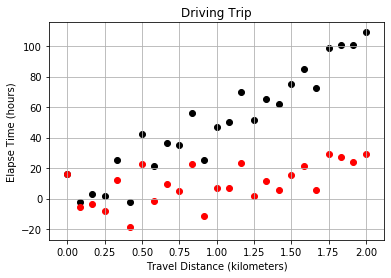In : # Build and fit two models, for columns distances1 and distances2 in df model_1 = ols(formula="distances1 ~ times", data=df).fit() model_2 = ols(formula="distances2 ~ times", data=df).fit() # Extract R-squared for each model, and the standard error for each slope se_1 = model_1.bse['times'] se_2 = model_2.bse['times'] rsquared_1 = model_1.rsquared rsquared_2 = model_2.rsquared # Print the results print('Model 1: SE = {:0.3f}, R-squared = {:0.3f}'.format(se_1, rsquared_1)) print('Model 2: SE = {:0.3f}, R-squared = {:0.3f}'.format(se_2, rsquared_2))  Model 1: SE = 3.694, R-squared = 0.898 Model 2: SE = 3.694, R-squared = 0.335  Notice that the standard error is the same for both models, but the r-squared changes. The uncertainty in the estimates of the model parameters is independent from R-squared because that uncertainty is being driven not by the linear trend, but by the inherent randomness in the data. This serves as a transition into looking at statistical inference in linear models. # Sample Statistics versus Population¶ In this exercise we will work with a generated population. We will construct a sample by drawing points at random from the population. We will compute the mean standard deviation of the sample taken from that population to test whether the sample is representative of the population. Our goal is to see where the sample statistics are the same or very close to the population statistics. ## Generate/Plot Data¶ In : sns.set(style="whitegrid", color_codes=True) # Seed random number generator np.random.seed(123) population = np.random.normal(99.98, 9.73, 310) sns.distplot(population, bins=25, color='r', kde=False);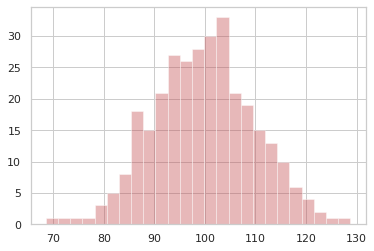In : # Compute the population statistics print("Population mean {:.1f}, stdev {:.2f}".format(population.mean(), population.std())) # Set random seed for reproducibility np.random.seed(42) # Construct a sample by randomly sampling 31 points from the population sample = np.random.choice(population, size=31) # Compare sample statistics to the population statistics print("Sample mean {:.1f}, stdev {:.2f}".format(sample.mean(), sample.std()))  Population mean 99.7, stdev 9.90 Sample mean 99.1, stdev 7.85  Notice that the sample statistics are similar to the population statistics, but not the identical. If you were to compute the len() of each array, it is very different, but the means are not that much different as you might expect. # Variation in Sample Statistics¶ If we create one sample of size=1000 by drawing that many points from a population. Then compute a sample statistic, such as the mean, a single value that summarizes the sample itself. If you repeat that sampling process num_samples=100 times, you get$100\$ samples. Computing the sample statistic, like the mean, for each of the different samples, will result in a distribution of values of the mean. The goal then is to compute the mean of the means and standard deviation of the means.
Here we will use population, num_samples, and num_pts, and note that the means and deviations arrays have been initialized to zero to give us containers to use for the for loop.
# Seed random number generator